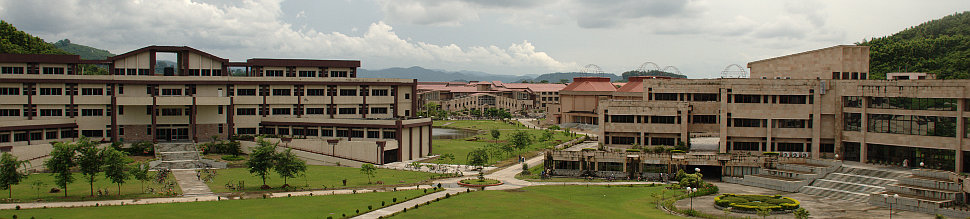••# Virtual laboratory Experience in Fluid and Thermal Sciences### Energy Loses in Pipe Flow

To determine the energy losses in pipe flow### Flow through Venturi Meter

To measure the volumetric flow rate of fluids.### Incompressible Flow through Nozzle and a Diffuser

Analysis of flow through a Nozzle and a Diffuser### Conduction Analysis of a Single Material Slab

Conduction analysis of Single Material Slab.### Conduction Analysis of a single Material Sphere

Conduction analysis of Single Material Sphere### Conduction Analysis of a single Material Cylinder

Conduction analysis of Single Material Cylinder.### Conduction Analysis of a Double Material Slab

Conduction analysis of Double Material Slab### Conduction Analysis of a Double Material Sphere

Conduction analysis of Double Material Sphere### Conduction Analysis of Double Material Cylinder

Conduction analysis of Double Material Cylinder.### To determine the overall heat transfer coefficient (U) in the parallel flow heat exchanger.

To determine the overall heat transfer coefficient (U) in the parallel flow heat exchanger.### To determine the overall heat transfer coefficient (U) in the counter flow heat exchanger.

To determine the overall heat transfer coefficient (U) in the counter flow heat exchanger.### To investigate the Lambert’s distance law.

To investigate the Lambert’s distance law### To investigate the Lambert’s direction law (cosine law).

To investigate the Lambert’s direction law (cosine law)